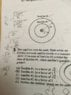# Physics: Circular motion and the orbital speeds of satellites

• YMMMA

## Homework Statement

Satellite 1 has mass m1 & radius R1 and satellite 2 has mass m2 and radius 2R1
Which satellite’s speed is greater?

Question and my attempt are shown in the attached file.

V= √GM/R

## The Attempt at a Solution

I am not sure if my steps are correct, but if there’s an easier solution, I need to know it.

#### Attachments

•image.jpg
41 KB · Views: 240
You are using the correct equations and you have the right answer. There is no easier solution if you are seeking a numerical answer for the ratio.

•berkeman, YMMMA and Janosh89
kuruman said:
You are using the correct equations and you have the right answer. There is no easier solution if you are seeking a numerical answer for the ratio.
Okay, then.